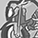App Development

Announcements
QlikWorld 2022, LIVE in Denver CO., May 16-19, 2022. REGISTER NOW TO RECEIVE EARLY BIRD PRICING
cancel
Showing results for
Did you mean:Contributor II

Compare selected month and previous month data

Hi! I have one problem, and it makes me crazy!

I need to compare the selected month and previous month data. For first, I need to get these data. I have tried to  get the sum of logged hours by these expressions (Moth_L this is a date):

Sum({\$<Month_L = {\$(=Max(Month_L) – 1)}>}  Log_Hours) - for previous month

OR

Sum({<Month_L = {">=\$(=Date(MonthStart(Max(MonthYear),-1),'MMM-YY'))<=\$(=Date(MonthStart(Max(MonthYear),-1),'MMM-YY'))"}>} Log_Hours)

and a few other expressions

But they return 0.

I think that's because I have selected Month_L in the filter. And when have added Month_L in the expression like an exception:

sum({\$<Month_L = {\$(=Max(Month_L)-1)}, Month_L=>}  Log_Hours)

And I get the sum working hours for all months.

So, how can I get the sum of hours by the previous month for comparing with the selected month?

1 Solution

Accepted SolutionsContributor II
Author

This expressions is correct:

Previous month:

Selected month:

Sum({< Log_Month = {">=\$(=MonthStart(Max(Log_Month)))<=\$(=MonthEnd(Max(Log_Month)))"}>} Log_Hours))Contributor II
Author

This expressions is correct:

Previous month: